# Algebra of measures

Jump to: navigation, search

measure algebra

The algebra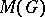of complex-valued regular Borel measures on a locally compact Abelian groupthat have bounded variation, with the ordinary linear operations and with convolution as multiplication (cf. Harmonic analysis, abstract). The convolutionof the measuresis completely defined by the condition that, for any continuous functiononwith compact support,If the total variation of a measure is taken as norm,becomes a commutative Banach algebra over the field of complex numbers. The algebra of measureshas a unit which is the-measure located at the zero of the group. The set of discrete measures contained informs a closed subalgebra.

To each functionwhich belongs to the group algebramay be assigned a corresponding measure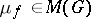in accordance with the rule(integral with respect to the Haar measure). The result is an isometric isomorphic imbedding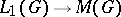. Under this imbedding the image is a closed ideal in.

The Fourier–Stieltjes transform of a measure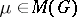is the function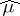on the dual groupdefined by the formula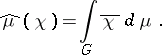Thenandif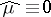. In particular,is an algebra without a radical.

If the groupis not discrete, then the structure of the measure algebrais extremely complicated: It is not symmetric and its space of maximal ideals has a number of pathological properties. For instance, this space contains infinite-dimensional analytic sets, and the naturally imbedded group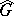in it is not dense even in the Shilov boundary. Nevertheless, the idempotent measures, i.e. measures for which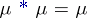, are known. Each idempotent measure is a finite integer combination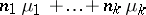, where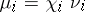, and whereis the Haar measure of a compact subgroup, andis a character. In the casethis means that a sequenceof zeros and ones is the Fourier–Stieltjes transform of some measure on the circle if and only ifdiffers from a periodic sequence by not more than a finite number of terms.

In the general case the theorem on idempotent measures can be naturally interpreted in terms of the cohomology spaces of dimension zero of the space of maximal ideals. A satisfactory description is also known for other cohomology groups of the space of maximal ideals of the measure algebra. This makes it possible to tell, in particular, if a logarithm of an invertible measure fromcan be taken (one-dimensional integral cohomology).